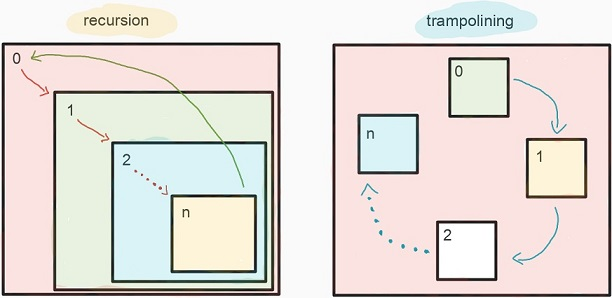## trampoline

Recursive tail call optimization Tail call optimization (or tail call elimination) allows recursive functions to re-use the stack

# trampoline

### Recursive tail call optimization

Tail call optimization (or tail call elimination) allows recursive functions to re-use the stack frame instead of creating new frames on every call.

Thanks to that an author of recursive function in tail position is not constrained by the stack size. More over such a function runs faster than the function without optimization, because calling a function doesn’t create a new stack frame which is time and resource consuming operation.

Let’s consider a function without a recursive call in tail position

In order to compute fact(n) we need first to compute fact(n – 1) and multiple a result by n. This process ends on a base case when we know that the fact(1) is just 1. This schema can be described formally using following recursive formula.

You can spot that the formula, and the implementation are almost identical, and this is a great advantage of using recursive functions. It makes code more readable and less error prone.

Let’s try to apply a fact with 5

Each call creates a new function frame on a stack (the call is mark by (..)) and because this call is not in a tail position a compiler can’t optimize this.

It turns out that fact can be slightly rewritten to have a recursive call in tail position. Take a look on fact2.

Here I introduced a helper function go which takes a role of stack safe iteration. Why this recursive call is stack safe? It is stack safe because the last recursive function call is the last instruction to do, and a compiler is aware of that. The compiler can utilize the current stack frame just by substituting the function parameters with the ones computed during the call. To have a better understanding we can simply assume that the compiler for optimization purpose rewrites fact2 to fact3.

fact3 uses imperative while loop to compute factorial which doesn’t introduce any new stack frames.

Limitation

Let’s consider a simple algorithm of checking if a given number is even or odd.

Even if the function calls are in tail positions, for n large enough we can notice stack overflow.

The sad true is that tail call optimization in the Scala compiler is limited to self-recursive methods.

Is there any way to deal with such constrained? It turns out that there is a solution in a form of simple technical trick.

Trampoline

A trampoline is a some kind of function interpreter. It can do two things:

• resume a suspended function call
• return a value computed by function to the caller side

A function running by a trampoline needs to be properly adjusted:

• if a function wants to make a call (might be recursive), it has to return the suspension instruction
• if a function wants to return a final result, it has to return an instruction describing the result

The interpretation process looks following:

• trampoline calls a function
• function returns the instruction
• trampoline interprets the instruction, and in case of suspension repeats the process
• in case of final result trampoline returns the value to its caller

All function calls are done via the trampoline. When a function has to call another function, instead of calling it directly, it provides the address of the function to be called and the arguments to the trampoline. This ensures that the stack does not grow and iteration can continue indefinitely. We can model instructions using simple ADT

Trampoline data type has two data constructors

• Return – indicates that the function wants to return a value to the caller side
• Suspend – suspends a function call (trampoline can resume)

Functions even and odd now can be rewritten respectively

The last piece of puzzle is an interpreter which is quite simple

Now we can try to run again the function even with large numbers, not being afraid of stack overflow